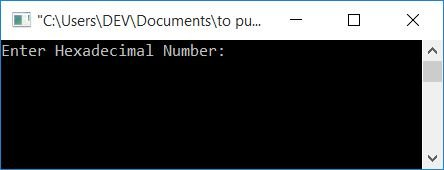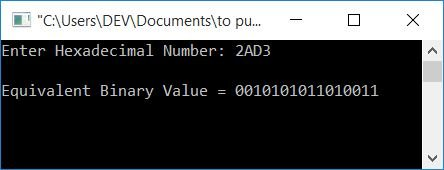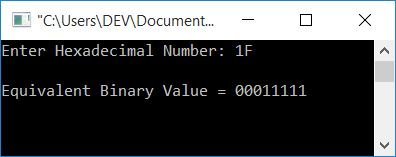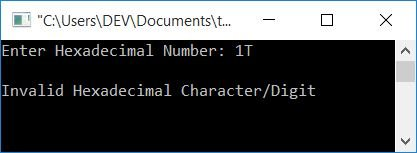# C Program to Convert Hexadecimal to Binary

In this article, you will learn and get code for the conversion of a given number from hexadecimal to binary.

But before going through the program, if you are not aware of

• Binary Number
• Hexadecimal to Binary Conversion Process

Then, refer to the step-by-step hexadecimal to binary conversion process. Now let's move on to the program given below.

## Hexadecimal to Binary in C

To convert a hexadecimal number to a binary number in C programming, you have to ask the user to enter the hexadecimal number to convert it into its equivalent binary number, as shown in the program given below. The question is: write a program in C that takes any number (in the hexadecimal system) as input and converts it into its equivalent binary value. The answer to this question is:

```#include<stdio.h>
#include<conio.h>
int main()
{
int i=0;
char hexdec;
gets(hexdec);
printf("\nEquivalent Binary Value = ");
while(hexdec[i])
{
switch(hexdec[i])
{
case '0':
printf("0000");
break;
case '1':
printf("0001");
break;
case '2':
printf("0010");
break;
case '3':
printf("0011");
break;
case '4':
printf("0100");
break;
case '5':
printf("0101");
break;
case '6':
printf("0110");
break;
case '7':
printf("0111");
break;
case '8':
printf("1000");
break;
case '9':
printf("1001");
break;
case 'A':
printf("1010");
break;
case 'a':
printf("1010");
break;
case 'B':
printf("1011");
break;
case 'b':
printf("1011");
break;
case 'C':
printf("1100");
break;
case 'c':
printf("1100");
break;
case 'D':
printf("1101");
break;
case 'd':
printf("1101");
break;
case 'E':
printf("1110");
break;
case 'e':
printf("1110");
break;
case 'F':
printf("1111");
break;
case 'f':
printf("1111");
break;
default:
printf("--Invalid Hex Digit (%c)--", hexdec[i]);
}
i++;
}
getch();
return 0;
}```

This program was compiled and executed in the Code::Blocks IDE. Here is the sample run. This is the initial snapshot of the sample run:Now supply any value (in the hexadecimal number system), say 2AD3, and press the ENTER key to see the equivalent value in the binary number system as shown in the snapshot given below:#### Program Explained

• declares a variable, say i, of the int type and initializes it with 0.
• Declares another char variable, say hexdec
• The maximum size of hexdec is defined as 11. That is, it can hold up to 10 characters. The last space is for a null-terminated character (\0).
• Now get the value of the hexadecimal number from the user (at run-time) using the gets() function.
• For example, suppose the user enters 2AD3 as a hexadecimal number. Then,
• 2 is saved at hexdec
• A is saved at hexdec
• D is saved at hexdec
• 3 is saved at hexdec
• So here we have created a while loop that runs until the last character of the given hexadecimal value.
• Inside the loop, we have used switch case to print the binary value for each and every hexadecimal character one by one.

### Hexadecimal to Binary in C using the strcat() Function

Now let's create another program that uses the string function strcat() to concatenate the string. Let's have a look at the program given below:

```#include<stdio.h>
#include<conio.h>
#include<string.h>
int main()
{
int i=0, chk=0;
char hexdec, binnum="";
gets(hexdec);
while(hexdec[i])
{
switch(hexdec[i])
{
case '0':
strcat(binnum, "0000");
break;
case '1':
strcat(binnum, "0001");
break;
case '2':
strcat(binnum, "0010");
break;
case '3':
strcat(binnum, "0011");
break;
case '4':
strcat(binnum, "0100");
break;
case '5':
strcat(binnum, "0101");
break;
case '6':
strcat(binnum, "0110");
break;
case '7':
strcat(binnum, "0111");
break;
case '8':
strcat(binnum, "1000");
break;
case '9':
strcat(binnum, "1001");
break;
case 'A':
strcat(binnum, "1010");
break;
case 'a':
strcat(binnum, "1010");
break;
case 'B':
strcat(binnum, "1011");
break;
case 'b':
strcat(binnum, "1011");
break;
case 'C':
strcat(binnum, "1100");
break;
case 'c':
strcat(binnum, "1100");
break;
case 'D':
strcat(binnum, "1101");
break;
case 'd':
strcat(binnum, "1101");
break;
case 'E':
strcat(binnum, "1110");
break;
case 'e':
strcat(binnum, "1110");
break;
case 'F':
strcat(binnum, "1111");
break;
case 'f':
strcat(binnum, "1111");
break;
default:
chk = 1;
break;
}
i++;
}
if(chk==0)
printf("\nEquivalent Binary Value = %s", binnum);
else
getch();
return 0;
}```

Here is the first sample run when the user enters any valid hexadecimal number, say 1F. Then the output produced by the above program will look like this:And here is another sample run, when the user enters any invalid hexadecimal digit, say 1T. Then the output produced by the above program will look like this:#### Program Explained

• Here, the binary equivalent of each and every hex digit gets concatenated one by one.
• And at last, we have checked and printed the equivalent binary value of the given hexadecimal value.
• Here we have checked whether the value of chk contains its original value or not.
• If it contains its original value, which is 0, then the default statement of switch case never runs.
• It means that no invalid hexadecimal character was entered by the user.
• And if chk does not hold its original value after exiting the while loop, then it means the default statement gets executed at once.
• So an invalid hexadecimal character was entered by the user.
• As a result, the program prints "Invalid Hexadecimal Character or Digit" as output.

#### The same program in different languages

C Quiz

« Previous Program Next Program »